## Induced Emf and Magnetic Flux

### Learning Objectives

By the end of this section, you will be able to:

• Calculate the flux of a uniform magnetic field through a loop of arbitrary orientation.
• Describe methods to produce an electromotive force (emf) with a magnetic field or magnet and a loop of wire.
The apparatus used by Faraday to demonstrate that magnetic fields can create currents is illustrated in Figure 1. When the switch is closed, a magnetic field is produced in the coil on the top part of the iron ring and transmitted to the coil on the bottom part of the ring. The galvanometer is used to detect any current induced in the coil on the bottom. It was found that each time the switch is closed, the galvanometer detects a current in one direction in the coil on the bottom. (You can also observe this in a physics lab.) Each time the switch is opened, the galvanometer detects a current in the opposite direction. Interestingly, if the switch remains closed or open for any length of time, there is no current through the galvanometer. Closing and opening the switch induces the current. It is the change in magnetic field that creates the current. More basic than the current that flows is the emfthat causes it. The current is a result of an emf induced by a changing magnetic field, whether or not there is a path for current to flow.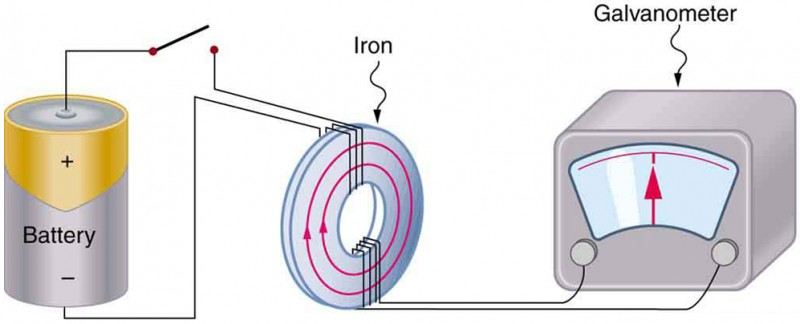Figure 1. Faraday’s apparatus for demonstrating that a magnetic field can produce a current. A change in the field produced by the top coil induces an emf and, hence, a current in the bottom coil. When the switch is opened and closed, the galvanometer registers currents in opposite directions. No current flows through the galvanometer when the switch remains closed or open.

An experiment easily performed and often done in physics labs is illustrated in Figure 2. An emf is induced in the coil when a bar magnet is pushed in and out of it. Emfs of opposite signs are produced by motion in opposite directions, and the emfs are also reversed by reversing poles. The same results are produced if the coil is moved rather than the magnet—it is the relative motion that is important. The faster the motion, the greater the emf, and there is no emf when the magnet is stationary relative to the coil.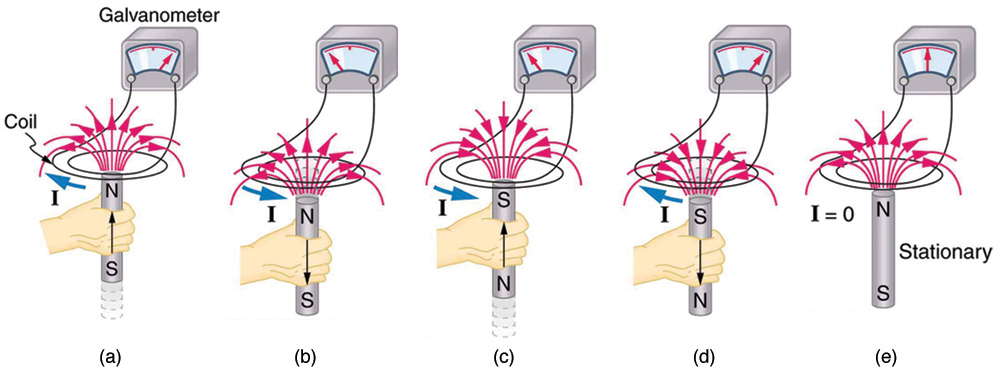Figure 2. Movement of a magnet relative to a coil produces emfs as shown. The same emfs are produced if the coil is moved relative to the magnet. The greater the speed, the greater the magnitude of the emf, and the emf is zero when there is no motion.

The method of inducing an emf used in most electric generators is shown in Figure 3. A coil is rotated in a magnetic field, producing an alternating current emf, which depends on rotation rate and other factors that will be explored in later sections. Note that the generator is remarkably similar in construction to a motor (another symmetry).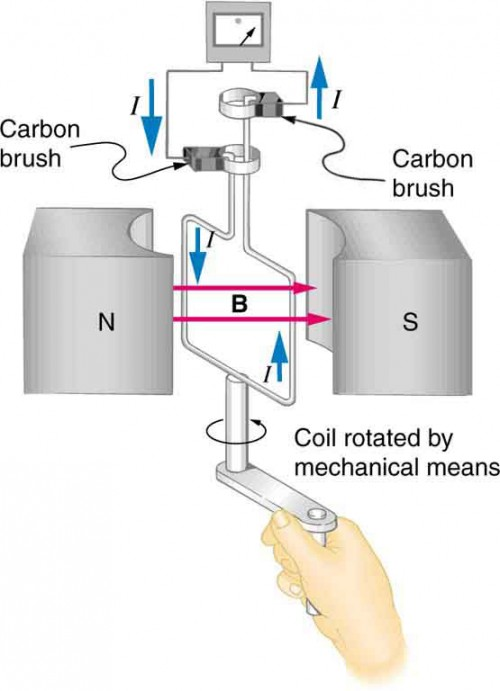Figure 3. Rotation of a coil in a magnetic field produces an emf. This is the basic construction of a generator, where work done to turn the coil is converted to electric energy. Note the generator is very similar in construction to a motor.

So we see that changing the magnitude or direction of a magnetic field produces an emf. Experiments revealed that there is a crucial quantity called the magnetic flux, Φ, given by

Φ BA cos θ,

where B is the magnetic field strength over an area A, at an angle θ with the perpendicular to the area as shown in Figure 5. Any change in magnetic flux Φ induces an emf. This process is defined to be electromagnetic induction. Units of magnetic flux Φ are T ⋅ m2. As seen in Figure 4, B cos θ = B, which is the component of B perpendicular to the area A. Thus magnetic flux is Φ BA, the product of the area and the component of the magnetic field perpendicular to it.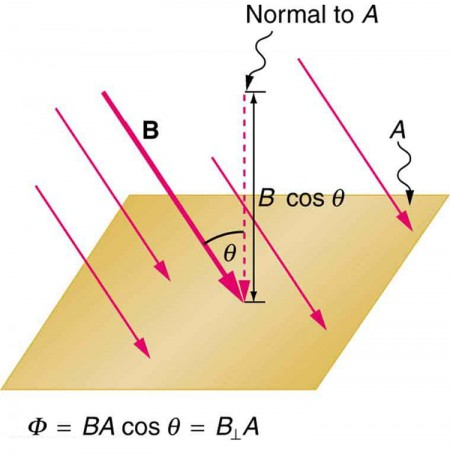Figure 4. Magnetic flux Φ is related to the magnetic field and the area over which it exists. The flux Φ = BA cos θ is related to induction; any change in Φ induces an emf.

All induction, including the examples given so far, arises from some change in magnetic flux Φ. For example, Faraday changed B and hence Φ when opening and closing the switch in his apparatus (shown in Figure 1). This is also true for the bar magnet and coil shown in Figure 2. When rotating the coil of a generator, the angle θ and, hence, Φ is changed. Just how great an emf and what direction it takes depend on the change in Φ and how rapidly the change is made, as examined in the next section.

## Section Summary

• The crucial quantity in induction is magnetic flux Φ, defined to be Φ BA cos θ, where B is the magnetic field strength over an area A at an angle θ with the perpendicular to the area.
• Units of magnetic flux Φ are ⋅ m2.
• Any change in magnetic flux Φ induces an emf—the process is defined to be electromagnetic induction.

### Conceptual Questions

1. How do the multiple-loop coils and iron ring in the version of Faraday’s apparatus shown in Figure 1 enhance the observation of induced emf?

2. When a magnet is thrust into a coil as in Figure 2(a), what is the direction of the force exerted by the coil on the magnet? Draw a diagram showing the direction of the current induced in the coil and the magnetic field it produces, to justify your response. How does the magnitude of the force depend on the resistance of the galvanometer?

3. Explain how magnetic flux can be zero when the magnetic field is not zero.

4. Is an emf induced in the coil in Figure 5 when it is stretched? If so, state why and give the direction of the induced current.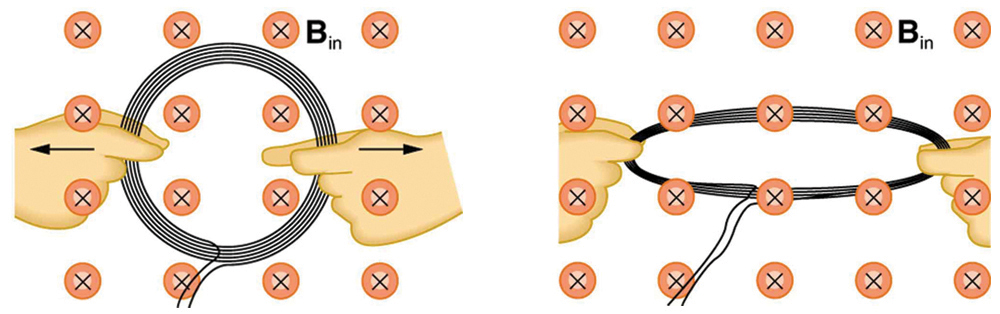Figure 5. A circular coil of wire is stretched in a magnetic field.

### Problems & Exercises

1. What is the value of the magnetic flux at coil 2 in Figure 6 due to coil 1?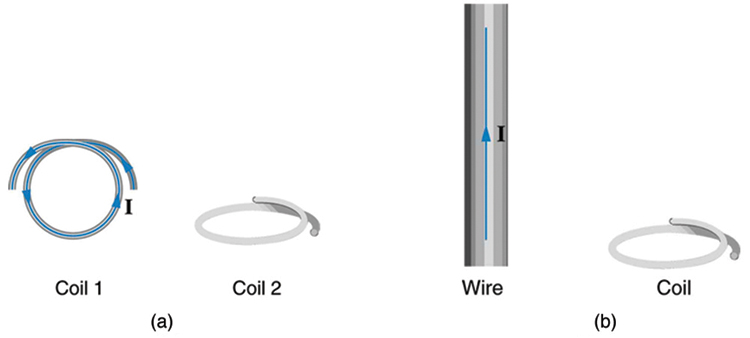Figure 6. (a) The planes of the two coils are perpendicular. (b) The wire is perpendicular to the plane of the coil.

2. What is the value of the magnetic flux through the coil in Figure 6(b) due to the wire?

## Glossary

magnetic flux:
the amount of magnetic field going through a particular area, calculated with Φ = BA cos θ where is the magnetic field strength over an area at an angle θ with the perpendicular to the area
electromagnetic induction:
the process of inducing an emf (voltage) with a change in magnetic flux

1. Zero